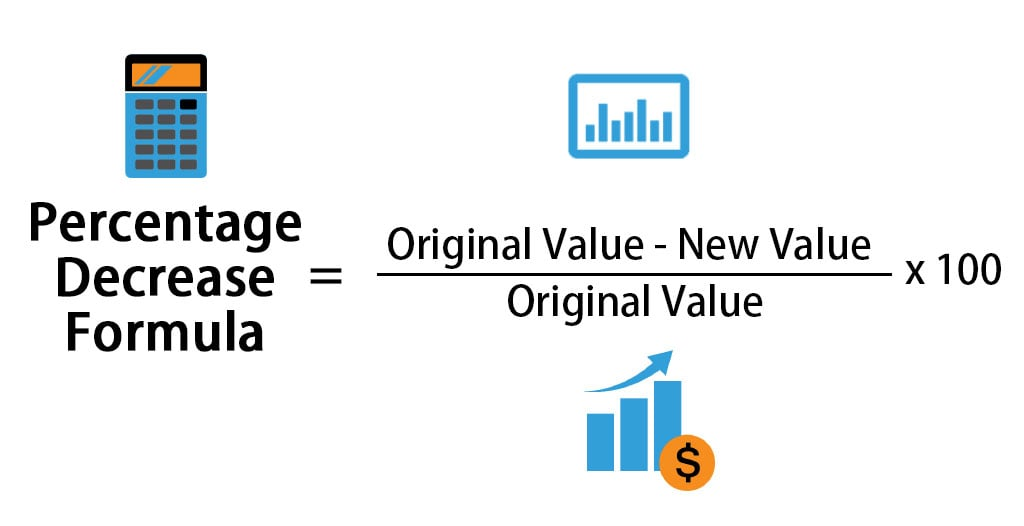# Percent Decrease Calculator

## Percentage Decrease Calculate

The Percentage decrease Calculator calculates the negative difference from one value to another in the form of a percentage. You have to enter starting value and final value to get the percentage decrease

### How to Calculate Percentage Decrease

The percentage Decrease Formula is

Percent decrease = [(original value – new value)/original value] * 100

• First value minus the second value
• Divide the result by the absolute value of the starting value
• Multiply by 100 to get a percentage decrease
• If the percentage is negative it means that is an increased value \### Percentage Decrease Calculator Example

You have a lamp with a 60-watt light bulb. Your lamp uses 60 watts of electricity per hour. You’re thinking of replacing the bulb with an LED light bulb that uses 8 watts of electricity per hour. What is the percentage decrease in the lamp’s hourly energy use if you switch to an LED light bulb?

Percentage Decrease = [ (Starting Value – Final Value) / |Starting Value| ] × 100

60 – 8 = 52
52 / 60 = 0.8667
0.8667 × 100 = 86.67%

Full Calculation Tool

Conversion Tool

### What is the percentage decrease from 100 to 10?

The percentage decrease from 100 to 10 is equal to 90%. Indeed, we get: `(100 - 10) / 100 = 0.9` and `0.9 × 100% = 90%`, as we claimed.

### What is the percentage decrease from 5000 to 1000?

The percentage decrease from 5000 to 1000 is equal to 80%. Indeed, we get: `(5000 - 1000) / 5000 = 0.8` and `0.8 × 100% = 80%`, as we claimed.